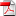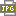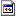Here are articles and thoughts in Finance, Physics and Mathematics, often in the intersection of these areas of science.

Before commencing I would like to say the following; As a mathematician and physicist, I often find that my work is lacking in some sense either from a physical intuitive point of view, or lacking mathematical standards of precision or rigor. I think that this is a burden that all of us working in the combination of these two sciences sometimes carry. And that does not mean that we should not strive to better ourselves. So be warned, there are omissions and sometimes mistakes in the following. I try to account for all such things in my erratas though.

Some of my achievements, things I have worked with and things I think about are;

1) Worked on the often mathematical foundations of gravity, such as proving a Maldacena Theorem for branes in a very general setting.

2) Suggested a lagrangean for gravity, which has critical dimension (this I define to be the dimension where the theory/action is scale invariant/dimensionless) 4 and is naively renormalizable in dimension 4. It implies all the standard features of gravity. See below for a article homepage.

3) Fruitless attempts; Made several ansatzes of a proof of Fermat's theorem which are intended to be short. I am working on this topic. (This is of course in Number theory) . There are many arguments and ideas in the literature on this topic and all of my attempts at a general theorem have been fruitless, albeit some special cases are easily proved. At least these fruitless attempts have been good as excercise. See below for ideas.

4) Invented the qualitative (risk and hedge) classification theory of financial options, by inventing the notions put-like and call-like, which together with the well know properties convexity and concavity suffice to say most things about the signs of (generalized) Greeks. These very notions also have applications in many other problems of mathematical finance, such as continuation domains of American options.

5) Worked on the Asian option pricing problem in mathematical finance.

6) Invented the stock crash and default theory of markets and companies. This can e.g. be used for valuation of credit risk.

7) Written a book on GUT and gravity, interrelating all branches of modern physics to a single whole; this leads to great advances in theoretical gravity physics. The name of the book is 'Noncommutativity and Origins of String Theory' under the pencil name 'Eric B Torbrand Dhrif'.

8) Investigating non-linear PDE's, such the parabolic Yang-Mill's, Ginzburg-Landau and phi-fourth equations. This is interesting in exact QFT. Also, I am thinking of how this relates to the issue of renormalization. Are there simple ways of renormalization once we know how to attack the exact field theory PDE's for some theory?? Partially, I think(know) that the answer is affirmative.

9) I have ventured into the possibility of a Gauge Theory and String Theory corresponce. My thoughts about dualities in String Theory, more explictly relating gauge theories and e.g type I and II strings, are accounted for in my book. This is related to an accurate description of moduli or Teichmuller space and it turns out that one has to look at a surface with punctures where the vertex operators are. You then have the right number of mass/moduli degrees of freedom to relate to gauge theory diagrams.

10) Proposing that supersymmetries might for N=1, 2 be identities and not symmetries, which I claimed was an application of the Pauli/Born interpretation of Quantum Theory. This is a radically different point of view in physics. This also explains why supersymmetric partners are not observed in nature.

11) Thinking about bound states in String Physics and also in QFT.

12) Thinking about holomorphic cobordism theory, this is related to Hartog's theorem and also Rouche's Theorem in the theory of several complex variables. Basically the ansatz or conjecture is that this boils down to a question of some sort of Dolbeault(d-bar) cohomology.  There may be some finer points to this.

13) Thinking about Neurosurgery, Nanosurgery and Nanogenetics.  I think that there is a promising future for these sciences which presently are in their cradle.

14) Invented a residue formula for Feynman diagrams which is a crossbreed which works well with the Diximier trace and is related to the Wodzicki residue. It keeps calculations nice, short and tidy-no more Feynman parameters and pages of calculus.

15) Thinking of models dual to String Theory, especially for the gravity sector-they look nice so far and are 'naively' renormalizable. These models are related to complex Stein manifolds, and display certain holography very naturally.

16) L^2 cohomology and the Novikov conjecture for Pontryagin classes and how these relate to dualities in string physics.

17) K-theory and how we describe multi-particle Hilbert spaces and composite systems in physics. This is also related to cohomology rings, Picard groups, Chern-Weil theory, etc.

18)Thom spaces-are they good candidates for holography in physics...the answers is yes. This relates to Stein spaces via theorems on the monodromy type of Stein Manfiolds. I touched this topic in a talk given at the Mittag-Leffler Institute, 2011-11-15.

19) Chiral splitting in both String Theory and Quantum Gravity, which makes the relevant theories much nicer to handle. This may be (is?) the missing ingredience in gravity. Actually this means that String Theory is equivalent to a 4-dimensional quantum field theory. The key to this is to differ between full-density theories(gravity, strings, statistical physics) and half-density theories(QFT, gauge theory) and how amplitudes form probablity densities to make physical predicitions. Since the square of  Gauge Theory/QFT S-matrix elements form probabilty densities we get exactly String Theory predictions. To 1-loop order this is very easy to prove. To multi-loop order you either patch and glue diagrams or use the path formulation of Dirac-Yang-Mills quantum field theory. Anyway, the point is to use this trick to make the calculations much less pathological.

20) How much can we make mass geometrical in physics? In String Theory I want to ask the following; Can a surface of arbitrary genus realise an arbitrary constant Ricci curvature/scalar upon embedding in a curved background- which would affect the curvature? Also there is the issue of piece-wise constant curvature, linked to theta function theory. By the great uniformization theorem in Riemann surface theory this is not so intrinsically or in a flat , say Euclidean, target space. Can the curvature of the background or target space affect this and is there 'quantum' curvature that does not follow the rules of classic curvature? I want this because I want to realise  my dream that all mass is geometry.  And if we say locally yes what does this mean globally? This is of course related to the issue of gauge-string dualities, momenta and masses in diagrams.

21) Pathology in geometrical models of Mathematical Physics; I think we should-often-work in a very general setting, not only using manifolds and varieties but actually embrace things like Riemann domains. These are generalizations of Riemann surfaces to several complex variables, basically a possibly pathological or degenerate fibration with at most  discrete and possibly variable fiber, with projection to a complex space of dimension greater or equal to two, or if you wish a sheaf with discrete stalk over any point on a complex space of higher dimension. Naturally the case of Riemann surfaces, which are Riemann domains of dimension one, is also relevant.  Note that in the applications we may permit these fibers or stalks to have coefficients in vector spaces, algebras, groups, etc.

22) The relation between string models-such as superstring theory-and their co-dimensional dual in e.g the standard D=10 space-time. This relates moduli wich are quite big on Riemann surfaces to moduli which are very small in magnitude- or however we may measure scarcity-  that is, this goes for the moduli space or Teichmuller space in D=8 dynamic space-time (Octonionic? Those moduli are not big!!) space-time. So the co-dimensional D=8 models are really quantum field theories with very little or no summation over moduli, yet still related to string physics. See the possible physical interpretation of Bott periodicities, such as in algebraic topology?

23) Moduli and mass spectra of intermediate interactions; it may be that the summation over  Teichmuller space is equivalent to a summation over intermediate particles in the diagrams of string or quantum field theory. However I am not sure that the sum measure is right.  If we see the measure as a e.g Källen-Lehmann spectral representation that we may have to infer the measure from empirical particle physics. This since the experimentally observed mass spectra are not corresponding to the simple mass spectra we have in standard string theory. Of course we have no such problems at very high energies, where the diagram amplitudes come out right anyway asymptoticaly or qualitatively. Note, however, that it is perfectly sensical that a non-massive particle diagram of some dimension, such as in string theory, can be seen as collections of massive particle diagrams in lower dimension(You can e.g think of Kaluza-Klein theory).

24) Measurment in String Physics/Quantum Gravity; e.g I propose that we artificially create physical systems that have a lot of particles in the same state. Such as Bose-Einstein supra-liquid Helium. And by this we 'magnify' these atoms so that we can measure very small String Theory/Quantum Gravity radiative corrections to Helium atomic spectra. The same line of reasoning goes for any system.  Either we may magnify it or make the measurment apparatus more delicate.  Bose-novas are another possibility for measurment of String Theory/Quantum Gravity predicitions, since initially we work with a Bose-Einstein condensate and then get a pulse of radiated particles, pending on your theory and sector, such as gravitons and photons. Maybe, just maybe, we can use that set-up to measure String Theory/Quantum Gravity corrections to bound states. This both in the lab or in the core of neutron stars.

25) On D-branes, gravity and supergravity, there seem to be relations that generalize my version(that is, the version I use and believe in) of supergravity to D-branes, actually matrix branes. This is very easy to see in the action and lagrangean density of this gravity. Please look on the article Last Version Gravity Matrix Branes etc below.

26) Worked on a algebraic approach to QFT. It uses Noncommutative Geometry and is supposed to make the calculus way more simple.

Below are some papers and my first book. Some of these articles are work in progress, and thus due for changes and corrections.creditII.pdf 174 KBEric Torbrand aug 2003.JPG 130 KBEricsBook15-10-10.pdf 2 MBErrata__Leaflet_Version_6.docx 13 KBLast Version GravityMatrixBranesJune2016.pdf 175 KBLast Version GravityQuantizedJanuary2019.pdf 400 KBLast Version P-brane Amplitudes and Diffeomorphism Symmetry June 2016.pdf 183 KBLast Version StringTheoryCorrelators.pdf 187 KBmono1.pdf 273 KB

The errata leaflet-please take care to look on the last version- is concerning my book ''Noncommutativity and Origins of String Theory".

I have four ansatzes to Fermat's Theorem. Disproving in quotient structures is ok as an idea of proof.  I do not know of anyone who has succeeded in using this trick, however. The second trick, disproving the equation for odd integers, x, y, z, and arbitrary positive integer powers greater than two and then using an interpolation is another ansatz(but not simple I think). I also have a third idea on a new tool for such integer equations, linking PDE's on tori and spectral representation theory to integer equations. One conclusion would be that if there is no solution to the corresponding PDE on a torus then there is no solution to the integer equation one looks at. Finally you could assume rational entries in two of the variables and then try to show that the remainding variable has to be irrational, and then understand that there are no integer solutions. If we are lucky, we may find a tool to approach this and other integer equations. So there is a motivation for research in this direction.

The general QFT diagram for a large set of theories is at;

http://www.m-hikari.com/astp/astp2013/astp5-8-2013/torbrandASTP5-8-2013-1.pdf

The Maldacena Theorem for branes is at (Please note that the Dirac operator on page 335 in the supergravity action is not the usual spinor Dirac operator, but what I call the vielbein Dirac operator. There is a supersymmetry in effective dimension or kinematical dimension 4 as well, and take care with different conventions for the gravity action.  There are numerical factors that differ, above all mutiplying the Ricci scalar curvature term. When you work in effective dimension or kinematical dimension 4 the operator Hamiltonian of vielbein gravity and the square spinor operator Hamiltonian coincide, which you can use to prove some important dualities in one single line.);

http://www.m-hikari.com/astp/astp2013/astp5-8-2013/torbrandASTP5-8-2013-2.pdf

The symmetry and self-consistency of P-branes, enforcing an Einstein condition, is at;

http://www.m-hikari.com/astp/astp2013/astp5-8-2013/torbrandASTP5-8-2013-3.pdf

A proposed quantum gravity (very much following the lines of reasoning in my book and published in Advanced Studies in Theoretical Physics with the title 'A More or Less Well-Behaved Quantum Gravity Lagrangean in Dimension 4?') is at

http://www.m-hikari.com/astp/astp2013/astp1-4-2013/torbrandASTP1-4-2013.pdf

I have vastly edited that article. The article LastVersionGravityQuantized etc, in the section above, is the last version of that article. It is much more thorough in this version.

The other articles that start with Last Version are in Brane Theory, String Theory and Quantum Field Theory; Gravity Matrix etc gives further thoughts on gravity and matrix branes. P-branes etc looks on branes and how they may behave in a Einstein setting for the brane, basically we find simpler ways of computing amplitudes. Also there is the issue of manifold surgery and how this works with branes. String theory correlators etc deals with possible novel expressions for propagators on brane sheets.

My string theory book is on Amazon at

http://www.amazon.com/s/ref=nb_sb_noss?url=search-alias%3Daps&field-keywords=eric%20torbrand%20dhrif

It may be that I will write a second book;

'Quantum Field Theory The Easy Way-Tricks of the trade'.